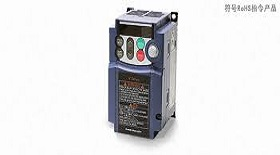## The other control modes

The third generation-vector control (VC) method.

Vector control variable frequency speed regulation is the stator current of asynchronous motor in the three-phase system Ia, Ib, Ic, through the three phase - two phase transformation, equivalent to two phase static coordinates system, ac current Ia1Ib1 again by pressing the rotor field-oriented rotation transform, the equivalent into synchronous rotating coordinates of dc current Im1, It1 (Im1 is equivalent to the excitation current of dc motor. It is equivalent to armature current that is proportional to torque), and then the control method of dc motor is imitated to obtain the control quantity of dc motor, and the control of asynchronous motor is realized through corresponding coordinate inverse transformation. In essence, the ac motor is equivalent to a dc motor, and the two components of the speed and magnetic field are independently controlled. By controlling the rotor flux and then decomposing the stator current, the torque and magnetic field are obtained. The proposed method of vector control has epoch-making significance. However, in practical application, because the rotor flux linkage is difficult to be accurately observed, the system characteristics are greatly affected by the motor parameters, and the vector rotation transformation used in the control process of the equivalent dc motor is complex, making the actual control effect difficult to achieve the ideal analysis results.

The fourth generation-direct torque control (DTC) method:

In 1985, professor DePenbrock of the ruhr university first proposed the direct torque control frequency conversion technology. This technology solves the shortage of vector control to a large extent, and develops rapidly with novel control ideas, concise system structure and excellent dynamic and static performance. The technology has been successfully applied to the high power ac transmission of electric locomotive traction. Direct torque control (DTC) directly analyzes the mathematical model of ac motor in the stator coordinate system. It does not need to be equivalent to dc motor, so it saves many complicated calculations in vector rotation transformation. It does not need to mimic the control of a dc motor, nor does it need to simplify the mathematical model of an ac motor for decoupling.